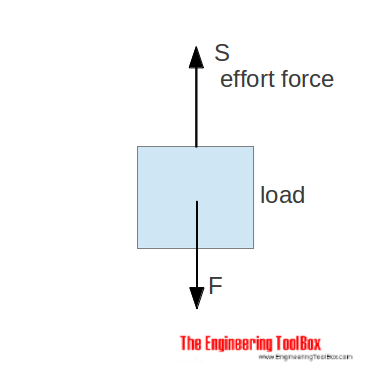Engineering ToolBox - Resources, Tools and Basic Information for Engineering and Design of Technical Applications!

# Movement Ratio

## Machines and movement ratio (velocity ratio).

A machine is a device that can change the magnitude and line of action of a force.Examples are

• pulley systems
• gear systems
• screw-jacks

A simple machine amplifies the input force (effort) to a larger output force (load).

### Movement Ratio

The movement ratio (or velocity ratio) of a machine is defined as the ratio of the distance moved by the effort to the distance moved by the load. Movement ratio can be expressed as

Mr = se / sl

= velocity ratio                         (1)

where

Mr = movement ratio

se = distance moved by the effort   (m, ft)

sl = distance moved by the load     (m, ft)

### Machine Efficiency

The efficiency of a simple machine is defined as the ratio of the force ratio to the movement ratio and can be expressed as

μ = Fr / Mr                       (2)

where

μ = machine efficiency

Fr = force ratio

## Related Topics

• ### Dynamics

Motion - velocity and acceleration, forces and torque.
• ### Mechanics

Forces, acceleration, displacement, vectors, motion, momentum, energy of objects and more.

## Related Documents

• ### Conn-Rod Mechanism

The connecting rod mechanism.
• ### Force Ratio

The force ratio is the load force versus the effort force.
• ### Motors - Speed vs. Pulley Diameter

Pulley (Sheave) selection charts for motors ranging 850 - 3450 rpm.
• ### Pulleys

Pulleys, blocks and tackles.
• ### Ratios and Proportions

The relative values between quantities - ratios and proportions.
• ### Screw Jack - Effort Force vs. Load

Screw jacks and effort forces.
• ### Toggle Joint

A toggle joint mechanism can be used to multiply force.

## Engineering ToolBox - SketchUp Extension - Online 3D modeling!

Add standard and customized parametric components - like flange beams, lumbers, piping, stairs and more - to your Sketchup model with the Engineering ToolBox - SketchUp Extension - enabled for use with older versions of the amazing SketchUp Make and the newer "up to date" SketchUp Pro . Add the Engineering ToolBox extension to your SketchUp Make/Pro from the Extension Warehouse !

We don't collect information from our users. More about

## Citation

• The Engineering ToolBox (2008). Movement Ratio. [online] Available at: https://www.engineeringtoolbox.com/movement-ratio-d_1295.html [Accessed Day Month Year].

Modify the access date according your visit.

9.19.12